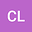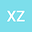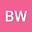Quasilinearized semiorthogonal B-spline wavelets method for solving multi-term nonlinear fractional order equations
•••• Can Liu,
• Xinming Zhang,
• Boying Wu
Can Liu
Harbin Institute of Technology Shenzhen
Author ProfileXinming Zhang
Harbin Institute of Technology (Shenzhen)
Author ProfileBoying Wu
Harbin Institute of Technology
Author Profile## Abstract

In the present article, we implement a new numerical scheme, the quasilinearization semiorthogonal B-spline wavelets method, combining the semiorthogonal B-spline wavelets collocation method with the quasilinearization method, for a class of the multi-term nonlinear fractional order equations. The fractional order equations contain Riemann-Liouville fractional integral operator and Caputo fractional differential operator. The quasilinearization method is firstly utilized to convert the multi-term nonlinear fractional order equation into a multi-term linear fractional order equation, which is solved by means of semiorthogonal B-spline wavelets subsequently. Herein, we investigate the operational matrix and the convergence of the proposed scheme. Several numerical results are given to confirm the accuracy and efficiency of our scheme.